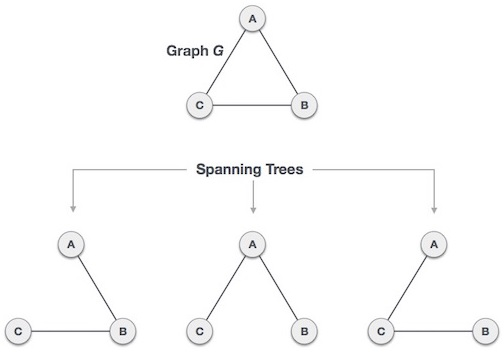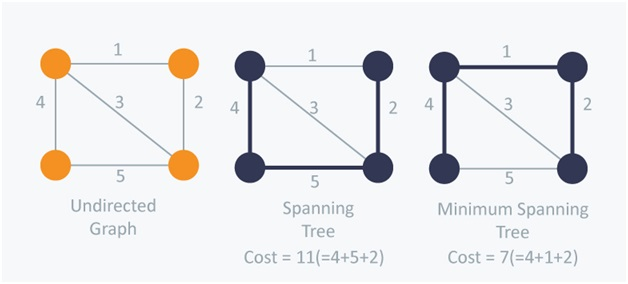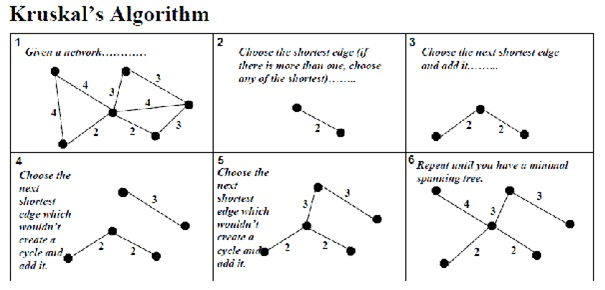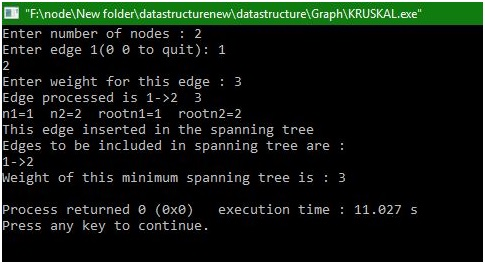# Creating a minimum spanning tree from Kruskal's algorithm

In this article, we will learn about spanning tree, minimum spanning tree and how to create a minimum spanning tree from Kruskal’s algorithm?
Submitted by Manu Jemini, on January 09, 2018

### 1) Spanning Tree

Spanning Tree is a subset of a graph, without any cycle. What this means is that if you can reach to node from a path A then path B is not needed, in fact, it can create a cycle, produces unnecessary weight.Image source: https://www.tutorialspoint.com/data_structures_algorithms/images/spanning_trees.jpg

### 2) Minimum Spanning Tree

Minimum Spanning Tree is a subset of a Graph, without any cycle. But it will be the best possible minimum cost spanning tree. What it means is, if you have to choose between two paths, minimum spanning tree always chooses the most efficient path.### 3) Kruskal’s Algorithm

Kruskal’s Algorithm is based on the concept of greedy algorithm. What it does is, it takes an edge with the minimum cost. The Algorithm will then take the second minimum cost edge. It will also make sure that the tree remains the spanning tree, in the end, we will have the minimum spanning tree ready.Image source: https://qph.ec.quoracdn.net/main-qimg-4e7075a1a6afe5273dd977a101ebf6a3

C program

```#include <stdio.h>
#include <malloc.h>
#define MAX 20

struct edge
{
int u;
int v;
int weight;
}*front = NULL;

int father[MAX]; /*Holds father of each node */
struct edge tree[MAX]; /* Will contain the edges of spanning tree */
int n;   /*Denotes total number of nodes in the graph */
int wt_tree=0; /*Weight of the spanning tree */
int count=0;    /* Denotes number of edges included in the tree */

/* Functions */
void make_tree();
void insert_tree(int i,int j,int wt);
void insert_pque(int i,int j,int wt);
struct edge *del_pque();

int create_graph()
{
int i,wt,max_edges,origin,destin;

printf("Enter number of nodes : ");
scanf("%d",&n);
max_edges=n*(n-1)/2;
for(i=1;i<=max_edges;i++)
{
printf("Enter edge %d(0 0 to quit): ",i);
scanf("%d %d",&origin,&destin);
if( (origin==0) && (destin==0) )
break;
printf("Enter weight for this edge : ");
scanf("%d",&wt);
if( origin > n || destin > n || origin<=0 || destin<=0)
{
printf("Invalid edge!\n");
i--;
}
else
insert_pque(origin,destin,wt);
}/*End of for*/
if(i<n-1)
{
printf("Spanning tree is not possible\n");
return 1;
}
}/*End of create_graph()*/

void make_tree()
{
struct edge *tmp;
int node1,node2,root_n1,root_n2;

while( count < n-1) /*Loop till n-1 edges included in the tree*/
{
tmp=del_pque();
node1=tmp->u;
node2=tmp->v;

printf("n1=%d  ",node1);
printf("n2=%d  ",node2);

while( node1 > 0)
{
root_n1=node1;
node1=father[node1];
}
while( node2 >0 )
{
root_n2=node2;
node2=father[node2];
}
printf("rootn1=%d  ",root_n1);
printf("rootn2=%d\n",root_n2);

if(root_n1!=root_n2)
{
insert_tree(tmp->u,tmp->v,tmp->weight);
wt_tree=wt_tree+tmp->weight;
father[root_n2]=root_n1;
}
}/*End of while*/
}/*End of make_tree()*/

/*Inserting an edge in the tree */
void insert_tree(int i,int j,int wt)
{
printf("This edge inserted in the spanning tree\n");
count++;
tree[count].u=i;
tree[count].v=j;
tree[count].weight=wt;
}/*End of insert_tree()*/

/*Inserting edges in the priority queue */
void insert_pque(int i,int j,int wt)
{
struct edge *tmp,*q;

tmp = (struct edge *)malloc(sizeof(struct edge) );
tmp->u=i;
tmp->v=j;
tmp->weight = wt;

/*Queue is empty or edge to be added has weight less than first edge*/
if( front == NULL || tmp->weight < front->weight )
{
front = tmp;
}
else
{
q = front;
}/*End of else*/
}/*End of insert_pque()*/

/*Deleting an edge from the priority queue*/
struct edge *del_pque()
{
struct edge *tmp;
tmp = front;
printf("Edge processed is %d->%d  %d\n",tmp->u,tmp->v,tmp->weight);
return tmp;
}/*End of del_pque()*/
main()
{
int i;

create_graph();
make_tree();
printf("Edges to be included in spanning tree are :\n");
for(i=1;i<=count;i++)
{
printf("%d->",tree[i].u);
printf("%d\n",tree[i].v);
}
printf("Weight of this minimum spanning tree is : %d\n", wt_tree);
}/*End of main()*/
```

Output